# Acid-Base Reactions: Introducing Ka and pKa

Last updated: February 20th, 2020 |

The Acidity Constant Ka (And Its Negative Logarithm pKa ) Is An Extremely Useful Measure Of Acidity

So last time we went through all the different trends that affect acidity. The bottom line is that any factor which stabilizes the conjugate base will result in increased acidity.

Now, it’s great to know trends.  It’s extremely important, in fact.

But what do you do when you want to compare the acidity of two molecules that aren’t connected by a trend?

For instance, resonance increases the acidity of alcohols, but by how much? More than you’d increase acidity by changing OH to SH? What if you added an electron withdrawing group?

It’s impossible to sort out all the opposing variables this way. Trends are only qualitative guides to this topic.

In order to answer this question we’ll need some hard data, and there’s only one way to get it in organic chemistry – through measurement.

And that’s what the acidity constant Ka (and its negative logarithm, pKa) are for.

## 1. The Acidity Constant Ka Represents The Equilibrium Constant For Dissociation Of An Acid Into Its Conjugate Base And A Proton

Let’s look at hydroiodic acid,  H–I. In solution (let’s use water)  H–I will protonate water to give H3O(+) and I(-). The reverse reaction also operates. [I (–) reacts with H3O(+) to give H-I back]. So this reaction is an equilibrium.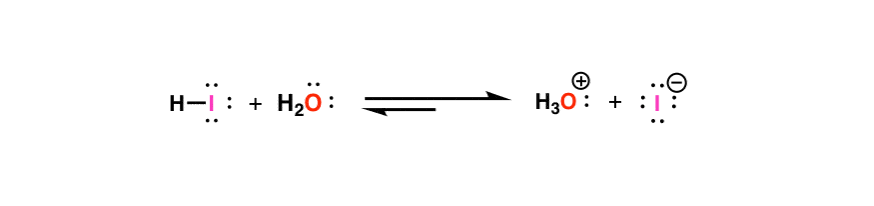Here’s the useful part. We can measure the equilibrium constant of this reaction, and that will tell us about the relative acidity of H-I. The specifics of how we can measure this are another story, but for our purposes, let me assure you that these types of equilibrium constants can be measured accurately.

For H-I, it turns out that the equilibrium constant for this reaction is about 10,000,000,000. That means for every molecule of HI, there are 10 billion molecules of I(-). In other words, H-I donates its proton very readily to give its conjugate base, which is a prime example of a strong acid.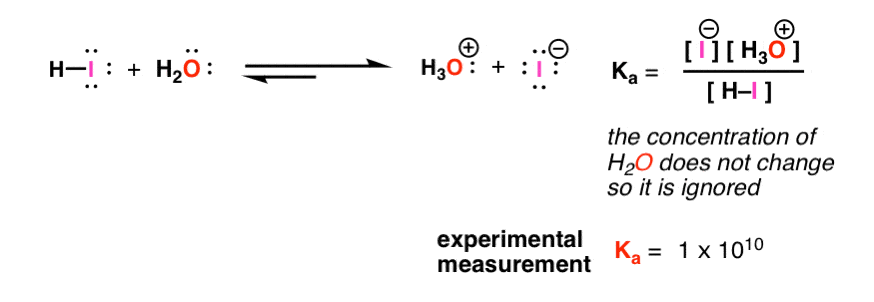So what? Well, we can do this not just for H-I, but for ANY species with a hydrogen. This equilibrium is the “ruler” by which we can measure any acid – by its equilibrium constant.*

We call this equilibrium constant Ka – the “acidity constant”.

## 2. Examples Of Acid Dissociation Constants Ka For A Strong Acid (HI), A Weak Acid (CH3OH), And An Extremely Weak Acid (CH4)

Let’s look at 3 examples. Note that these are somewhat oversimplified in that the solvent has been left out.*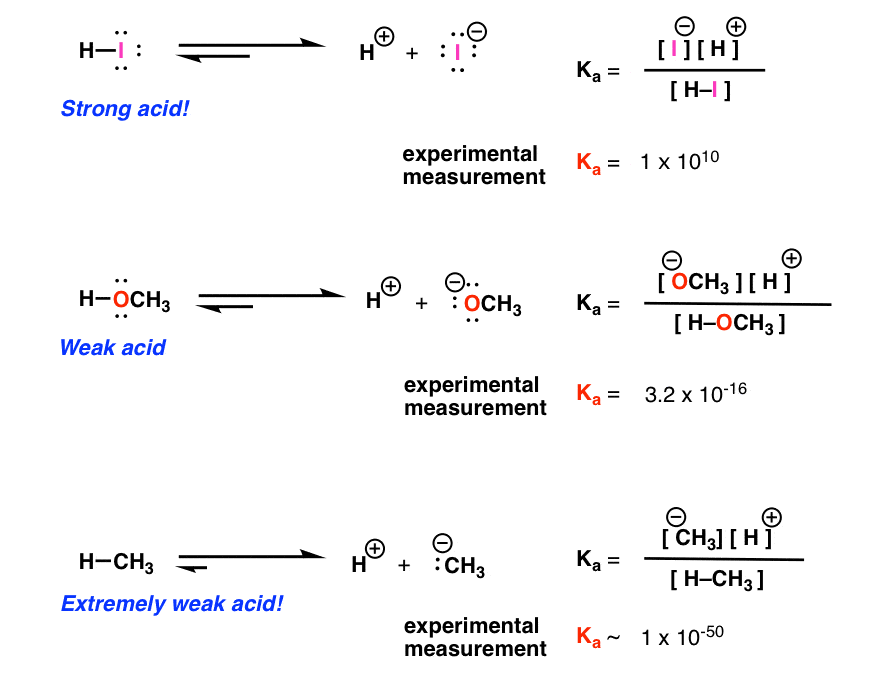Now comparing acidity between numbers with lots of exponents after them is not the most convenient way to do things. So instead, we’ve taken to using a logarithmic scale. These are common – the Richter scale is logarithmic, for instance – an 8.0 magnitude quake is 10 times more powerful than a 7.0 magnitude quake.

## 3. The Negative Logarithm Of Ka, “pKa” Is A Far More Convenient Measure Of Acidity Than Ka

For acidity, the number we use is called “pKa”. It’s obtained by taking the logarithm of the acidity constant Ka, and we arbitrarily decide to multiply it by negative one [the vast majority of Ka values are less than 1, and we’re generally more comfortable dealing with positive numbers].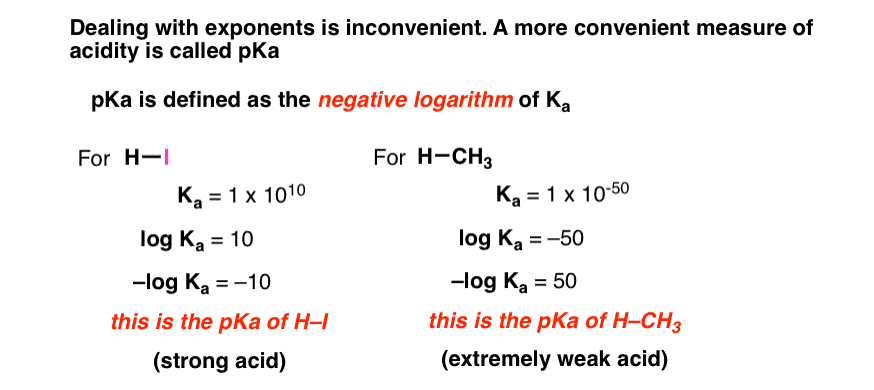So for H-I, the pKa is –10 (representing a very strong acid), that for methanol is 15 (a weak acid), and for methane, it’s 50 (an extremely weak acid).

## 4. The pKa Scale Encompasses Over Sixty Orders Of Magnitude

That scale comprises 60 orders of magnitude. That’s a huge number!!!!!

To give you an idea of the scale, of pKa, this is the range of the smallest value for length (the Planck length, 10–35 meters), to the width of the known universe (about 1026  meters). This website has a phenomenal animation of this.

Anyhow, these measurements have been done for thousands of different molecules now. The result is a big table that allows us to compare the acidity of all kinds of different functional groups. Here’s an example of a pKa table from a previous post.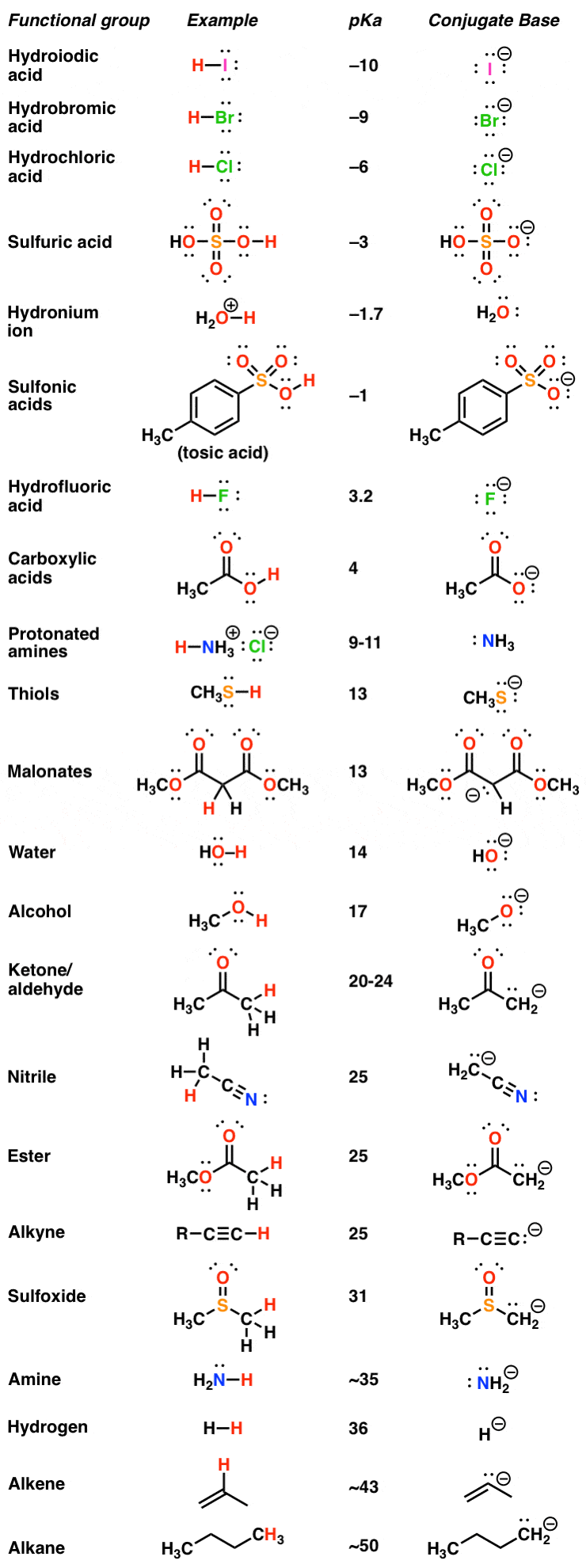The pKa table is your friend. In one document, it gives you information on the scope and magnitude of a wide range of chemical behavior – the strongest of the strong acids, and the weakest of the weak acids. And since the stronger the acid, the weaker the conjugate base, it also provides information about basicity.

Next Post: How to Use A pKa Table

## Notes

* N.B. The choice of solvent for these measurements is important due to the “leveling effect”. For instance  Water is commonly used as a solvent for molecules with a pKa value less than zero and no greater than about 15, since there can be no stronger acid in water than H3O(+) and no stronger base than HO(-). To get some of the more extreme values other solvents must be used, such as dimethyl sulfoxide (DMSO). If you end up in a more advanced organic chemistry course, look at some of these values with a harder eye through that lens. Strongly recommended – Evans’ pKa table gives values in H2O and DMSO. And there is the heroic efforts of Hans Reich at Wisconsin in compiling pKa values.

## Comment section

### 7 thoughts on “Acid-Base Reactions: Introducing Ka and pKa”

1.BRSM says:

Good post! Looks like you forgot to put in the link when you say ‘This website has a phenomenal animation of this’.

2.Alyx vance says:

There’s something I don’t quite grasp in the HI reaction to give I-.
If the Ka value is 10^10, then doesn’t that mean that for every molecule of HI, the product of concentrations [I-] and [H3O+], instead of just [I-]? So shouldn’t there be the square root of 10^10 molecules of I-?

3.Abbi Z. says:

The link for the Evan’s pka table no longer exists. I searched a little and I think this is the link you want now: http://evans.rc.fas.harvard.edu/pdf/evans_pKa_table.pdf

1.James Ashenhurst says:

Yes, thank you!

4.Sourav says:

I have a doubt:
when a polar protic solvent is used as a solvent for its conjugate base, will the conjugate base be the strongest base/nucleophile in that solvent?
Thanks!

1.James Ashenhurst says:

Yes, this is known as the “leveling effect”. The strongest base possible in a solvent is the conjugate base of that solvent. So the strongest base that can exist in H2O is HO- (the conjugate base of water) since if one was to add a stronger base (e.g. NaNH2) the base would immediately deprotonate the solvent, generating its conjugate acid (NH3) and the conjugate base of the solvent (HO- )

5.Sourav says:

Ah, Ohkay!
Thank you so much!

This site uses Akismet to reduce spam. Learn how your comment data is processed.